Tips & Tricks: Coding-Decoding

# Tips & Tricks: Coding-Decoding - Tips & Tricks for Government Exams - Bank Exams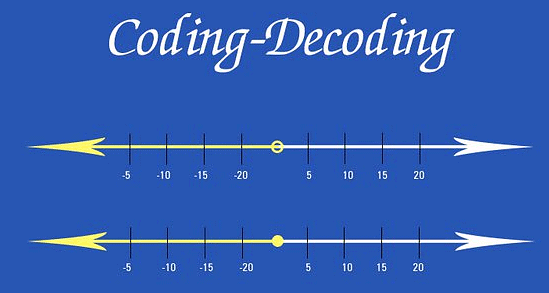## Coding Decoding Shortcut Tricks

First of all we should know the positioning (in numerical value) and opposite of all alphabets. The below given diagram explain itself: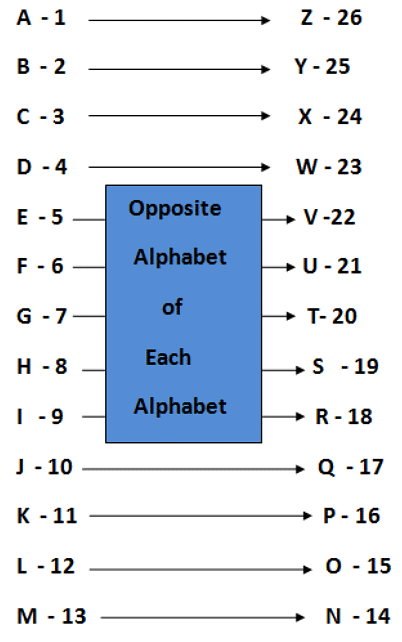(Above given diagram shows the numerical values of different alphabets as well as particular alphabet's opposite alphabets e.g. Numeric value of F is 6 and opposite of F is U and U's numeric value is 21 and so on).

## Examples

Example 1: If in a certain language "NIL" is written as "MOHJKM", then how will "BOND" be written in that language?
(a) ACNPOMCE
(b) ANCPMOCE
(c) ACNPMOCE
(d) ACNPMEOC
(e) None of The Above
Ans:
(c)
Solution: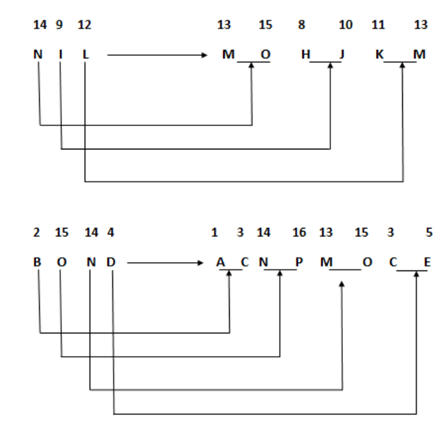Trick: Each letter is coded with its left and right letters in English alphabet manner is used.

Example 2: If in a certain language, "TOP" is written as "OQNPSU", then how will "SUN" be coded in that language?
(a) MOVTRT
(b) MOTVRT
(c) MOVTTR
(d) MOVRTT
(e) None of The Above
Ans:
(b)
Solution: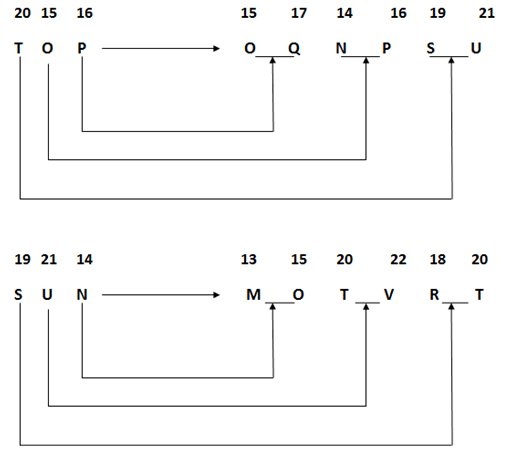Trick: Each letter is coded with its left and right letters in English alphabet manner is used.

Example 3: If "PKROK" is coded as 72962 and KRRPK as "29972", then how can "QLSAZ" be coded?
(a) 45716
(b) 74315
(c) 91523
(d) 51430
(e) None of The Above
Ans:
(d)
Solution: P - 7
K - 2
R - 9
O - 6
K - 2
(From above it shows that P is coded as 7, K is coded as 2, R is coded as 9 and O is coded as 6 that means code of "QLSAZ" does not have either 7, 2, 9, 6 in options. Hence, required code will be 51430, which is given in Option D). In simple words, we can say that the options which does not contain either of 7, 2, 9 and 6 is answer.

Example 4: If in a certain code language "SIMILAR" is written as "IZORNRH", then how will "BECTOR" be written in that language?
(a) ILGXYV
(b) ILGXVY
(c) ILXGYV
(d) ILXYVG
(e) None of The Above
Ans:
(b)
Solution: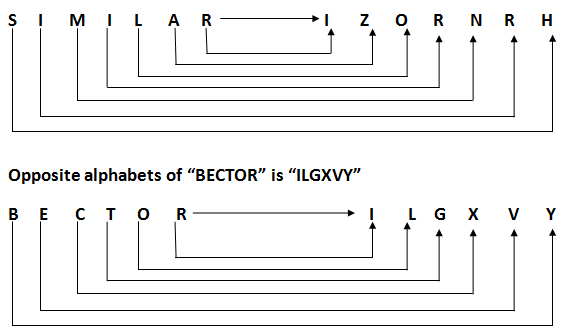Trick of Opposite Alphabets is used.

Example 5: In a certain code language "TIGER" is written as "2097518", then how will "TENDULKAR" be written in that language?
(a) 205144212156212
(b) 205144221111182
(c) 205144211211118
(d) 205144211532522
(e) None of The Above
Ans:
(c)
Solution: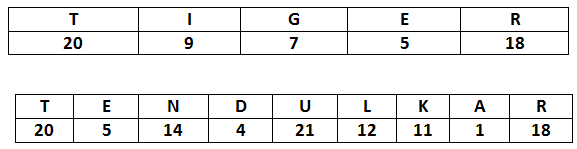Trick of Alphabets in numerical value is used.

The document Tips & Tricks: Coding-Decoding | Tips & Tricks for Government Exams - Bank Exams is a part of the Bank Exams Course Tips & Tricks for Government Exams.
All you need of Bank Exams at this link: Bank Exams

## Tips & Tricks for Government Exams

66 videos|65 docs

## FAQs on Tips & Tricks: Coding-Decoding - Tips & Tricks for Government Exams - Bank Exams

 1. What is coding-decoding in the context of competitive exams?Ans. Coding-decoding is a problem-solving technique in competitive exams where a series of letters, numbers, or symbols are given, and based on certain rules, the examinee is required to decipher the pattern or rule and find the correct answer.
 2. How can one improve their coding-decoding skills for exams?Ans. To improve coding-decoding skills for exams, one can practice solving various coding-decoding puzzles regularly. Reading books or online resources that provide different coding-decoding patterns and techniques can also be helpful. Additionally, participating in mock tests and solving previous year's question papers can enhance problem-solving abilities in this area.
 3. What are some common types of coding-decoding patterns in exams?Ans. In exams, some common types of coding-decoding patterns include letter shifting, number substitution, symbol rotation, mirror image coding, and alphabetical series coding. These patterns require logical thinking and understanding of the given rules to decode the given information accurately.
 4. Are there any shortcuts or tricks to solve coding-decoding questions quickly?Ans. Yes, there are certain shortcuts or tricks that can help solve coding-decoding questions quickly. For example, identifying recurring patterns or observing the relationship between the given letters or numbers can provide valuable clues. Additionally, practicing mental calculations and developing a systematic approach to problem-solving can significantly improve efficiency in solving coding-decoding questions.
 5. How can one approach complex coding-decoding questions in exams?Ans. Complex coding-decoding questions in exams can be approached by breaking down the given information into smaller parts and analyzing them individually. It is essential to identify the rules or patterns governing each element and use logical reasoning to decode the entire sequence. Practice and familiarity with different coding-decoding patterns can enhance the ability to tackle complex questions effectively.

## Tips & Tricks for Government Exams

66 videos|65 docsExplore Courses for Bank Exams examSignup to see your scores go up within 7 days! Learn & Practice with 1000+ FREE Notes, Videos & Tests.
10M+ students study on EduRev
Track your progress, build streaks, highlight & save important lessons and more!
Related Searches

,

,

,

,

,

,

,

,

,

,

,

,

,

,

,

,

,

,

,

,

,

;